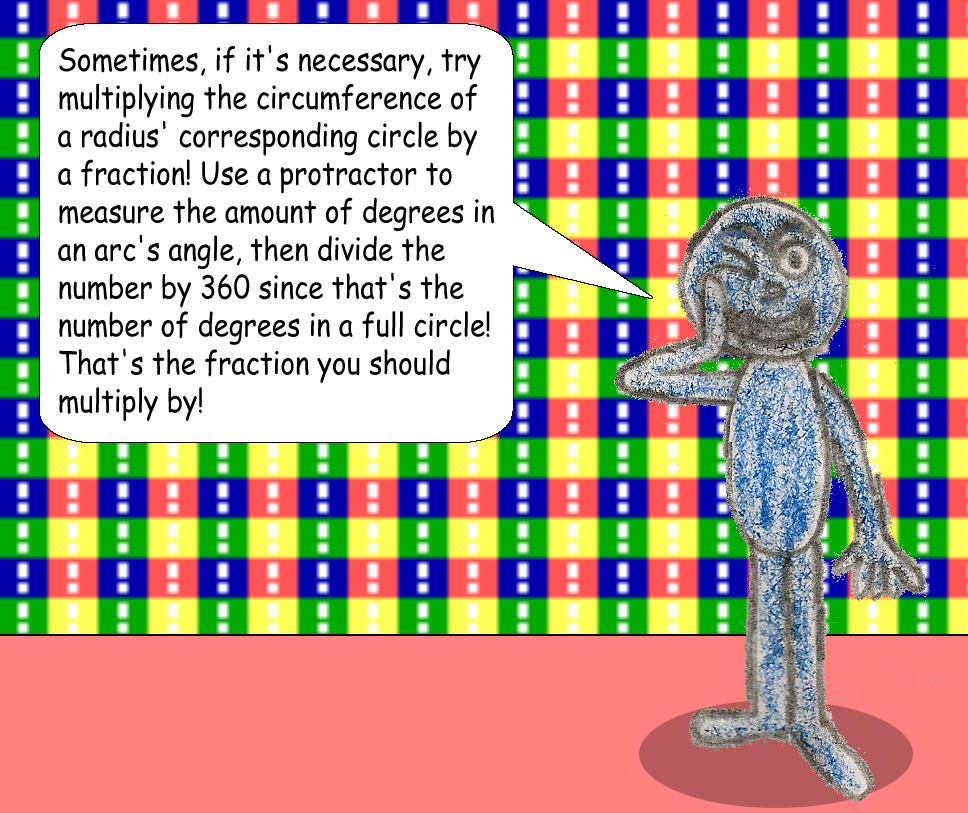# The Formula for the Circumference of a Spiral

C = 2 * Pi * R = Pi * D

## This is the well-known formula for the circumfence of a circle, and it can partially be used to figure out the circumference of a spiral!## which is the circumference of the spiral!

### The formula is simple enough for spirals that are perfectly circular, but what if the spiral happens to be more like an oval?### Of course, Derek C. Jr.'s tip will also work for the circumference of an oval!

To make things simpler, you can multiply the radius of an arc by the angle of the same arc in radians.